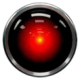b.d -3 years ago 97
Java Question

# Using Spring @Pattern validation. What regular expression would let max 7 digits with up to 3 decimal places

`^\d{0,7}(\.\d{0,3})?\$`
is not allowed due to Invalid escape sequenceTodd

Assuming you are defining a String like this:

``````String pattern = "^\d{0,7}(\.\d{0,3})?\$";
``````

The `\` inside the String needs to be escaped in Java.

``````"^\\d{0,7}(\\.\\d{0,3})?\$"